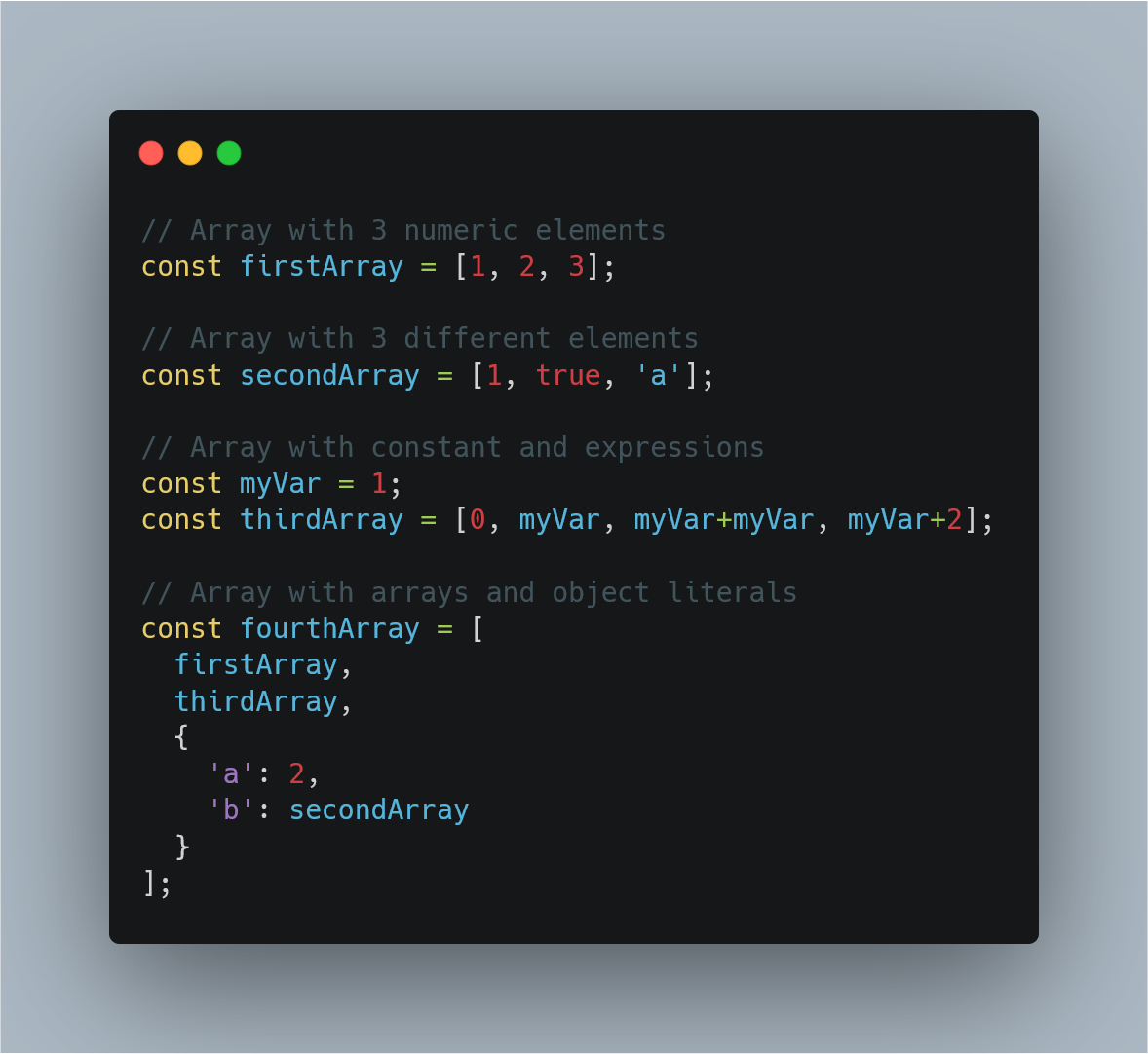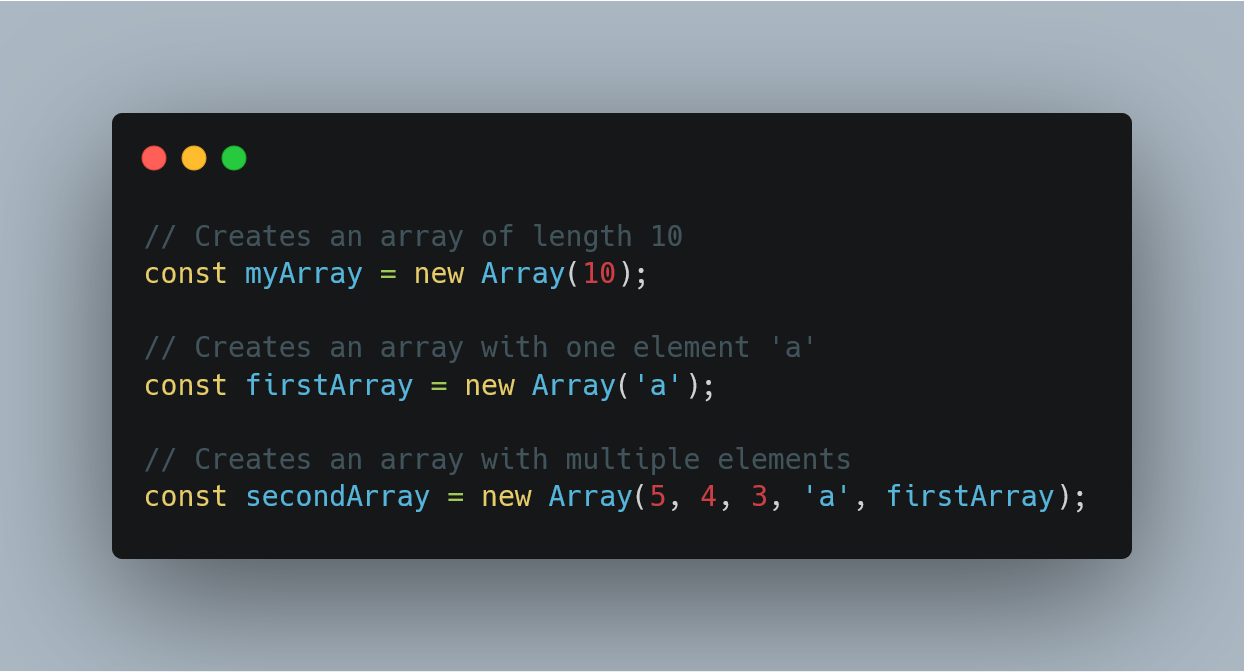1596111600

# JavaScript Array Some Example | Array.prototype.some()

Javascript array some() function tests whether some item in the array passes the test implemented by the provided function. The some() method is the JavaScript extension to the ECMA-262 standard; as such, it may not be present in other implementations of the standard.

## JavaScript Array Some Example

JavaScript array some() is the inbuilt method that tests whether at least one item in the array passes the test implemented by a provided function. It returns the Boolean value.

The some() method in JavaScript executes the function once for each element present in the array:

1. If it finds an array element where the function returns a true value, then some() function returns true (and it does not check the remaining values).
2. Otherwise, it returns false.

### Syntax

``````array.some(function(currentValue, index, arr), thisValue)
``````

### Parameters

JavaScript some() function takes the first parameter as a function that takes the following parameters.

1. currentValue: It is Required. The value of the current element.
2. index: It is Optional. The array index of the current element.
3. arr: It is Optional. The array object the current element belongs to.

The function is the required parameter.

It takes thisValue as a second parameter, which is optional. It is the value to be passed to the function to be used as its “this” value. If the thisValue parameter is empty, the value “undefined” will be given as its “this” value.

### Return Value

Javascript some() method returns true if the callback function returns a truthy value for at least one element in the array. Otherwise, it returns false.

### Example

Write the following code inside the new file. Let’s call it the app.js file.

``````// app.js

let dark = [
100,
90,
80,
];

console.log(dark.some(x => x > 90));
``````

#### Output

``````true
``````

In this example, we defined an array and then check if the array contains a single item that satisfies the condition, and if it does, then returns true otherwise, it returns false.

#javascript #array #programming

## Buddha Community1600410360

## Javascript Array From Example | Array.prototype.from()

Javascript array from() is an inbuilt function that creates a new, shallow-copied array instance from an array-like object or iterable object.

The Array .from() lets you develop Arrays from the array-like objects (objects with a length property and indexed items) or  iterable objects ( objects where you can get its items, such as Map and  Set).

The Array from() function was introduced in ECMAScript 2015.

### Javascript Array From Example

Array.from() method in Javascript is used to creates a new  array instance from a given array. If you pass a  string to the Array.from() function, then, in that case, every alphabet of the string is converted to an element of the new array instance. In the case of integer values, a new array instance simply takes the elements of the given Array.

The syntax of the Array.from() method is the following.

#### Syntax

``````Array.from(arrayLike[, mapFn[, thisArg]])
``````

#javascript #ecmascript #javascript array from #array.prototype.from1600506180

## Javascript Array Shift Example | Array.prototype.shift()

Javascript array shift() is an inbuilt function that removes the first item from an array and returns that deleted item. The shift() method changes the length of the array on which we are calling the shift() method. Javascript Array Shift method is not  pure function as it directly modifies the  array.

### Javascript Array Shift Example

Javascript shift() method removes the item at the zeroeth index and shifts the values at consecutive indexes down, then returns that removed value.

If we want to remove the last item of an array, use the  Javascript pop() method.

If the length property is 0,  undefined is returned.

The syntax for shift() method is the following.

``````array.shift()
``````

An array element can be a  string, a number, an  array, a boolean, or any other  object types that are allowed in the Javascript array. Let us take a simple example.

#javascript #array.prototype.shift #javascript pop #javascript shift1624388400

## JavaScript Arrays Tutorial. DO NOT MISS!!!

Learn JavaScript Arrays

📺 The video in this post was made by Programming with Mosh
The origin of the article: https://www.youtube.com/watch?v=oigfaZ5ApsM&list=PLTjRvDozrdlxEIuOBZkMAK5uiqp8rHUax&index=4
🔥 If you’re a beginner. I believe the article below will be useful to you ☞ What You Should Know Before Investing in Cryptocurrency - For Beginner
⭐ ⭐ ⭐The project is of interest to the community. Join to Get free ‘GEEK coin’ (GEEKCASH coin)!
Thanks for visiting and watching! Please don’t forget to leave a like, comment and share!

#arrays #javascript #javascript arrays #javascript arrays tutorial1602154740

## Fill and Filter in Array in JavaScript

By the word Array methods, I mean the inbuilt array functions, which might be helpful for us in so many ways. So why not just explore and make use of them, to boost our productivity.

Let’s see them together one by one with some amazing examples.

### Array.fill():

The `_fill()_` method changes all elements in an array to a static value, from a start index (default `_0_`) to an end index (default `_array.length_`). It returns the modified array.

In simple words, it’s gonna fill the elements of the array with whatever sets of params, you pass in it. Mostly we pass three params, each param stands with some meaning. The first param value: what value you want to fill, second value: start range of index(inclusive), and third value: end range of index(exclusive). Imagine you are going to apply this method on some date, so that how its gonna look like eg: array.fill(‘Some date’, start date, end date).

``````NOTE: Start range is inclusive and end range is exclusive.
``````

Let’s understand this in the below example-

``````//declare array
var testArray = [2,4,6,8,10,12,14];

console.log(testArray.fill("A"));
``````

When you run this code, you gonna see all the elements of `testArray` will be replaced by `'A'` like `[“A”,"A","A","A","A","A","A"]`.

#javascript-tips #array-methods #javascript-development #javascript #arrays1597470780

## Introduction

Arrays are a structure common to all programming languages so knowing what they are and having a firm grasp on what you’re able to accomplish with Arrays will take you a long way in your journey as a software developer. The code examples I share in this post will be in JavaScript but the concepts are common among all languages. What you learn here can easily be translated to any other language you work with.

In this post I’ll be covering how to perform the create, read update and delete operations using arrays, some common functions that come with the Array prototype and also how to implement them.

## What is an Array

Before we jump into the juicy bits of Arrays, lets quickly gloss over what they are. Arrays

• are a fundamental data type in JavaScript
• are an ordered collection of values called **elements **that are stored at and accessed via an index
• are untyped, meaning that the elements of an array could be of different types. This allows us to create complex arrays such as an array of objects or even an array of arrays (multidimensional arrays)
• can have elements that are constants or expressions
• have a property called length that tells you the number of elements in the array
• inherit properties from `Array.prototype` that includes a wide variety useful functions that can be called from arrays or `array-like` objects

## CRUD operations using Arrays

If you’re not familiar with the term CRUD it stands for Create, Read, Update and Delete. In this section we’ll go through each one of these operations and cover different ways you can perform each one.

### Creating Arrays

There are several ways you can create an Array but the most common ways are by using

• the Array literal syntax
• the Array constructor i.e. `new Array()`

Lets take a look at each one with examples

### Array literal

The array literal is the most common way of creating an array. It uses the square brackets as a notion of a container followed by comma separated values inside the square brackets. The following examples show how to use the array literal syntax and how arrays are untyped i.e. can contain elements of different types.Examples of untyped arrays in JavaScript created with the array literal syntax.

### Array constructor

Another way to create an array is through the Array constructor.

``````const myArray = new Array();
``````

Using the Array constructor, as shown above, is the same as creating an array with the array literal syntax. i.e.

``````// The following two lines behave exactly the same way i.e. both create an empty arrays

const myArray = new Array();
const myOtherArray = [];
``````

The array constructor, however, is able to receive arguments that allow it to behave in different ways depending on the number and type of arguments passed to it.

• You can pass a single numeric argument which creates an array of the specified length. This option is mostly used when you know how many elements you’ll be placing in the array
``````const myArray = new Array(5);
``````

Note: If you want to define the array with a specified size, as shown above, the argument passed must be a numeric value. Any other type would be considered as the first element that’ll be placed in the array.

• Or you can pass two or more arguments or a non-numeric argument to place the values inside the array. This works the same way as shown in the array literal examples.Examples of arrays created by using the Array constructor in JavaScript

As stated earlier, these two ways are the most common ways of creating arrays that you’ll see and use 99% of the time. There are a few other ways but we won’t dive deep into how they work. They are

• the spread operator `const someArray = […someOtherArray]`
• the static method `Array.of()`
• and the static method `Array.from()`

#javascript #web-development #javascript-tips #javascript-development #javascript-arrays #sql